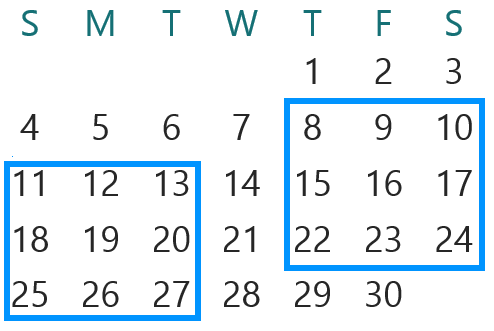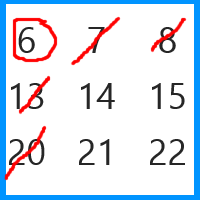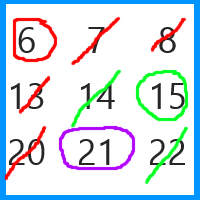#### You may also like### Consecutive Numbers

An investigation involving adding and subtracting sets of consecutive numbers. Lots to find out, lots to explore.Make a set of numbers that use all the digits from 1 to 9, once and once only. Add them up. The result is divisible by 9. Add each of the digits in the new number. What is their sum? Now try some other possibilities for yourself!# Calendar Capers

##### Age 11 to 14 Challenge Level:

You will need a calendar for this problem; you can print one off here.

Choose any three by three square of dates on a calendar page.

Add the numbers in the four corners.Try a few examples. What do you notice?

Can you explain why this happens?

Now try adding the numbers in each row, column and diagonal that passes through the centre number.

What do you notice?
Can you explain your results this time?

Now try adding the numbers in the top and bottom rows, and the left and right columns.

What do you notice?

Finally...

Circle any number on the top row and put a line through the other numbers that are in the same row and column as your circled number.

Repeat this for one of the remaining numbers in the second row.

You should now have just one number left on the bottom row; circle it.

Here is an example:Find the total for the three numbers circled. What do you notice?

What if you choose different numbers to circle?
What if you choose a different three by three square?